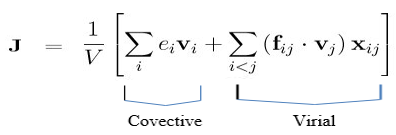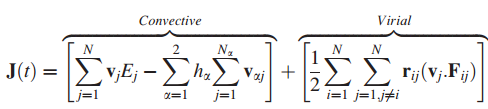# Reg: Green-Kubo to measure thermal conductivity of the nanofluid

Dear all,

The Green-Kubo formula in LAMMPS is used to determine the thermal conductivity of a single component system, i.e. single phase homogeneous system. example Ar.For the multi-component system (nanofluid), the enthalpy term has to be subtracted from the convective term to get significant results according to the attached paper. The new GK formula for the multi component system:The problem I am facing is how to incorporate this enthalpy term in the formula to calculate the correct thermal conductivity of the multi-component system. Please suggest me the method to proceed in the problem. Any kind of help is appreciated. Thanks

Dear all,

The Green-Kubo formula in LAMMPS is used to determine the thermal
conductivity of a single component system, i.e. single phase homogeneous
system. example Ar.
!image.png|396x135
For the multi-component system (nanofluid), the enthalpy term has to be
subtracted from the convective term to get significant results according to
the attached paper. The new GK formula for the multi component system:

The problem I am facing is how to incorporate this enthalpy term in the
formula to calculate the correct thermal conductivity of the
multi-component system. Please suggest me the method to proceed in the
problem. Any kind of help is appreciated. Thanks

​i am not aware of a flag or compute in LAMMPS that can do this for you.

axel.​